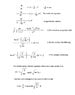# Air resistance equation derivation

• Us477

#### Us477

I would like to derive the air resistance equation showed in the attached photo. A little while ago, I tried asking at a different forum, however, the users were very experienced and omitted several stages of the derivation, and thereby made the interpretation difficult as I am new to math, but extremely eager to learn. I hope someone can help me illuminate each step of the derivation process, this would be a great help for my future studies, which are independant - I am not attending school at the moment. I have spent much time with this problem and I appreciate all help

Thanks from Denmark :)

#### Attachments

•Equation.jpg
19.6 KB · Views: 971

When you say you are new to math, how new is that? Have you studied integral calculus and differential equations? Without knowledge of some advanced math, this forum is not suitable for detailed explanation of the derivation you seek.

I think that that second-to-last equation where you finally get v(t) to the left hand side follows from:

$tanh ( arctanh( \sqrt{ \frac{\rho}{g} }v ) ) = \sqrt{ \frac{\rho}{g} } v = tanh( \sqrt{ \frac{\rho}{g} } (gt + c) )$
Which follows from the given fact that $\rho = \frac{k}{m}$.

From there it's a matter of rearraning, and using what I assume will be some given physical constraint which tells us that c is 0 or may be taken to be 0.

To do the final step, it's not immediately clear to me how to integrate that, I would consult an integral table for integrands containing tanh(z) .

Hope that helps somewhat.

Note also that $tanh^{-1}(z)$ denotes the inverse hyperbolic tangent, or arctanh(z), and not the reciprocal of tanh(z). That would be coth(z).

EDIT: Yes, I would also agree that you should make yourself comfortable with basic calculus and differential equations. There are a number of free reading courses online for both, and you should be able to find good references using Google or the search function. There are many skills and tricks in Calculus that are very important to being able to manipulate physical equations.

Last edited:
Yes, I have studied calculus and differential equations, and solved many different types of problems, but I am unable to solve this specific problem. When I wrote "new" I meant that I had not studied differential equations for several years

Thanks Ocifer, now I am one step further, will update the picture I uploaded later today.

Last edited: